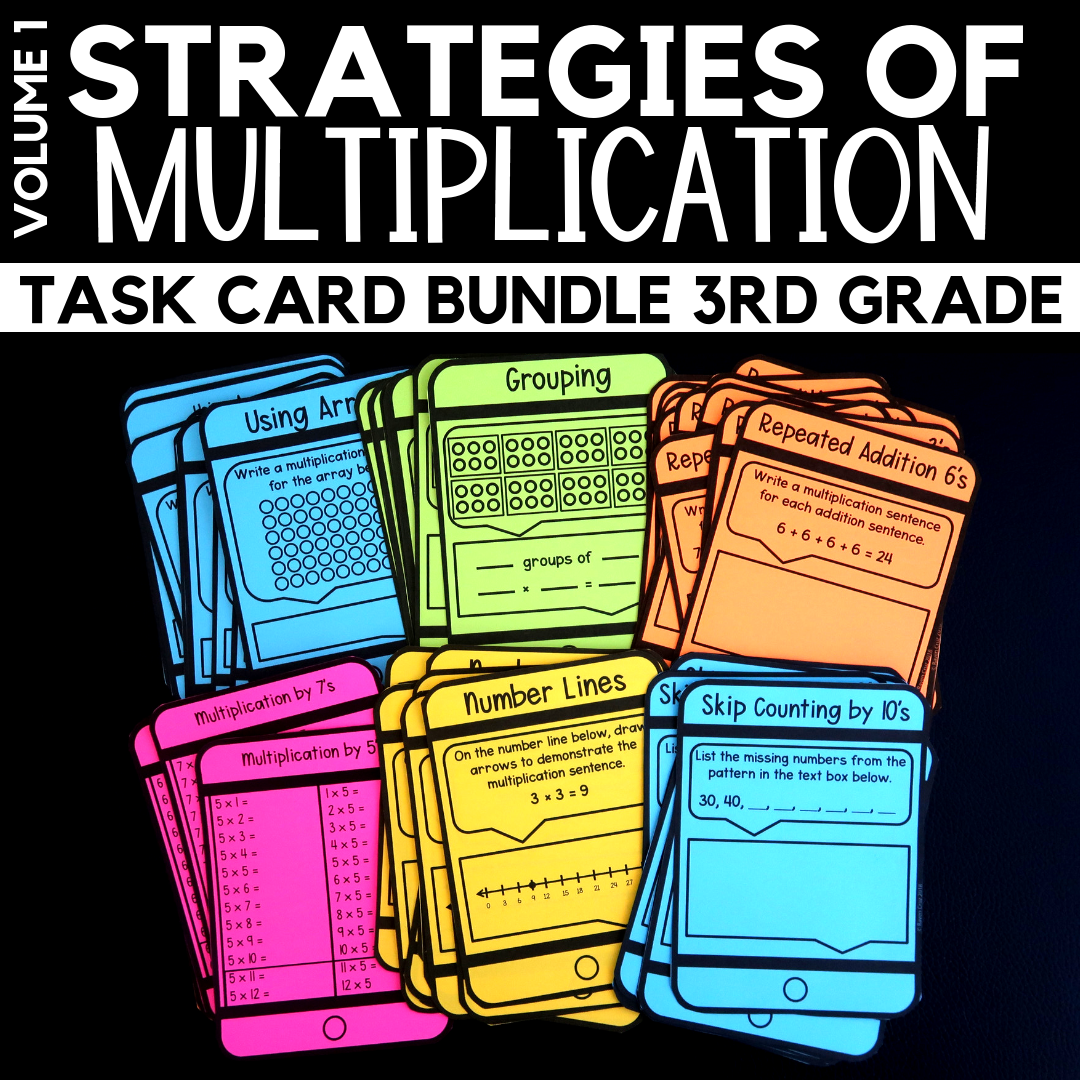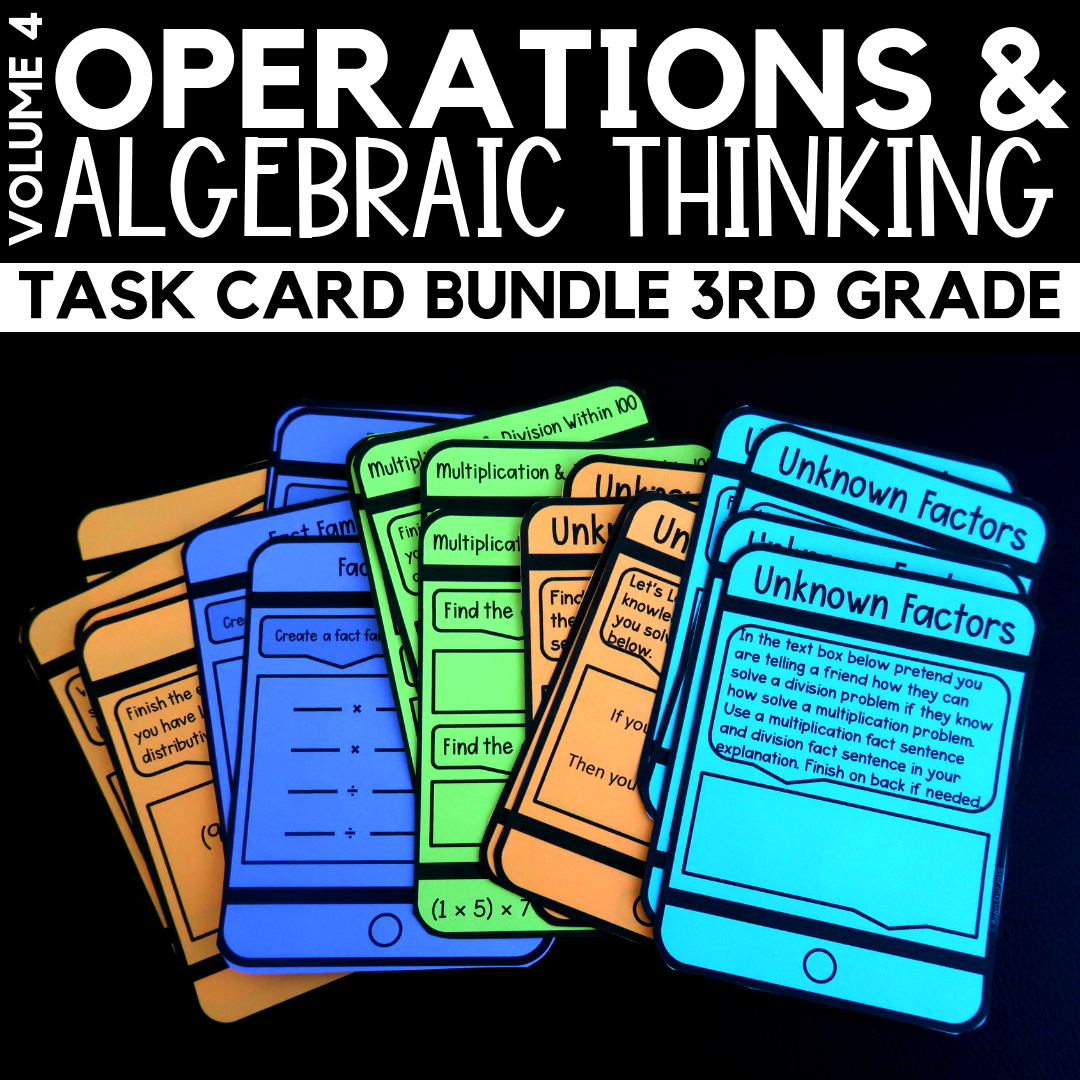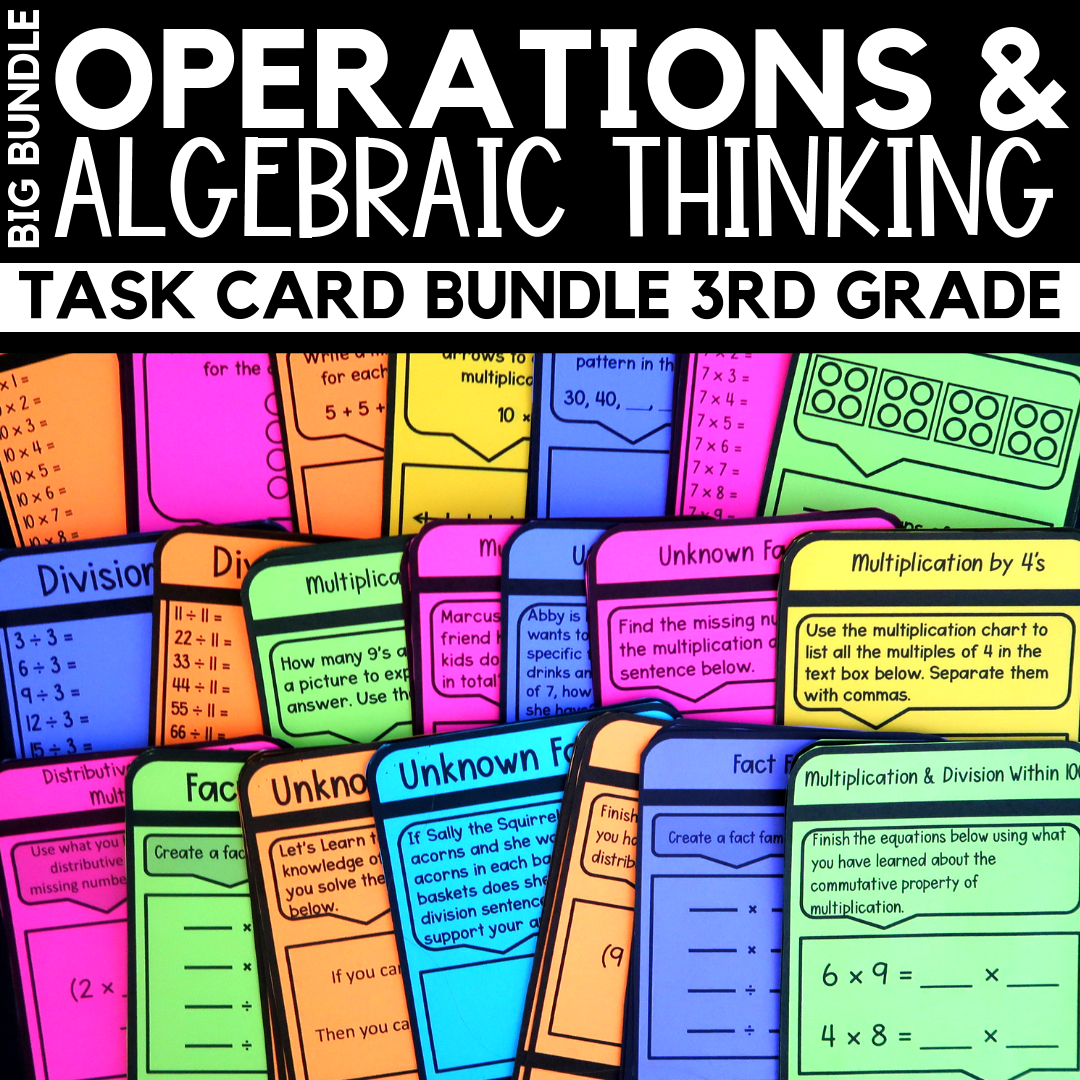Multiplication Strategies - Raven Cruz

# Multiplication Strategies

Five multiplication strategies that I use when teaching multiplication include; equal groups or grouping, arrays, repeated addition, skip counting, or number lines. The strategies help students with their multiplication fluency, as well as break down a

#### Multiplication by Grouping

The first multiplication strategy is learning multiplication by grouping is the process of separating objects into equal groups. Most commonly when using equal groups, repeated addition is also used. This method is one of the most straightforward ways for students to comprehend the strategy of multiplication by grouping.

As you can see in the example below there are 3 equal groups of 2 cookies. Students can use repeated addition to solve a multiplication problem like the one below.

In the classroom teachers can use manipulative such as; erasers, counters, small blocks, or even drawing on dry erase boards to practice the strategy of grouping and repeated addition.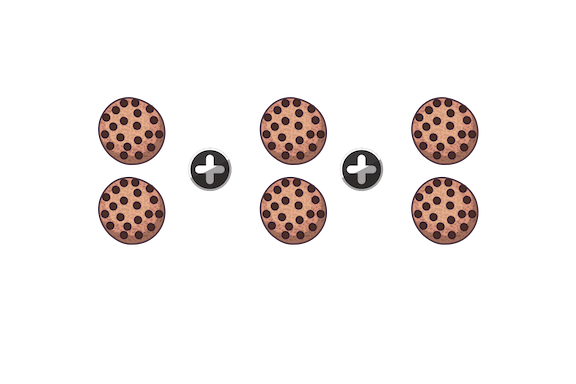2 + 2 + 2 = 6

The second multiplication strategy is repeated addition. We touched on using repeated addition with grouping above when we grouped 2 cookies in groups of three. Both learning using grouping and learning using arrays are complimentary to each other.

3 + 3 + 3 + 3 + 3 + 3 = 18

#### Multiplication by Arrays

The third multiplication strategy is arrays. Arrays are an arrangement of objects arranged in rows and columns.

The arrangement of cookies shown below shows the systematic way to introduce arrays.  The student will first learn a 1 × 4 array, 2 × 4 array, 3 × 4 array, 4 × 4 array, and so on... A larger arrangement of objects can also be broken down into arrays to preform many different types of multiplication, such as properties of multiplication.

Making arrays in the classroom can be fun AND engaging for students. Using colorful objects, such as; erasers, counters, and blocks to build arrays can keep all types of learners engaged and ready to learn.1 × 42 × 43 × 44 × 4

#### Multiplication by Number Lines

Fourth, there is learning multiplication by number lines. Students will take "jumps" on the number line. These jumps help students solve  multiplication problems by skip counting.

Number lines are an exceptional way to teach number sense as well.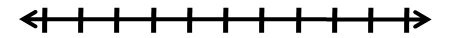2  4  6  8 10.....

#### Multiplication by Skip Counting

Last, learning multiplication by number lines uses the next strategy - skip counting. Skip counting is not just for providing students a way to count faster, it also helps them solve multiplication problems.

Starting at 0 students count in intervals until reaching the desired number. Students can also point out the pattern being used when skip counting.

0, 5, 10, 15, 20, 25, 30...

## Resources to Teach Multiplication

Check out the many resources in my Teachers Pay Teachers store that help teachers provide FUN ways to teach multiplication. A few of my recommendations are listed below. The resources are broken down into volumes to help teach your students systematically.